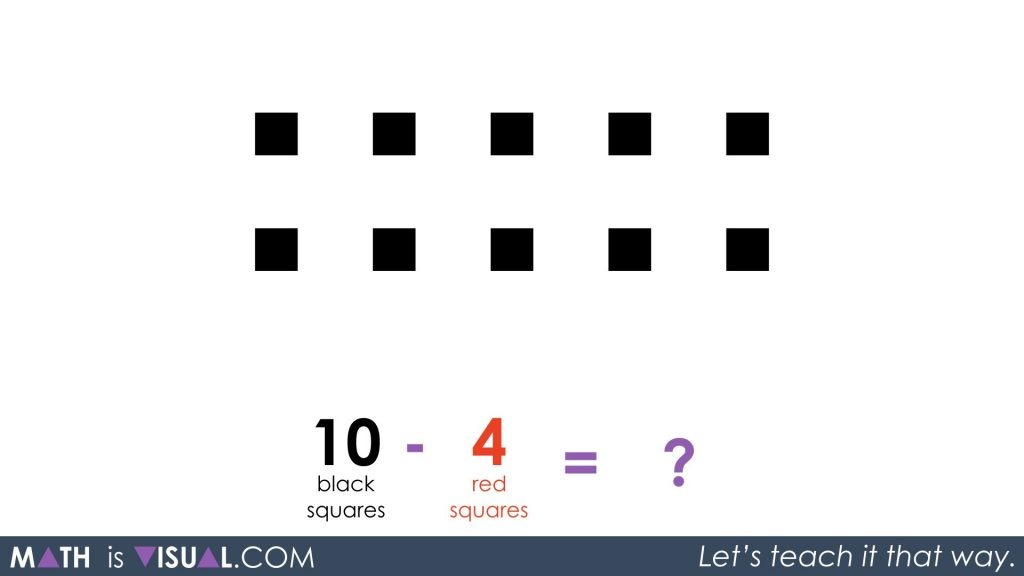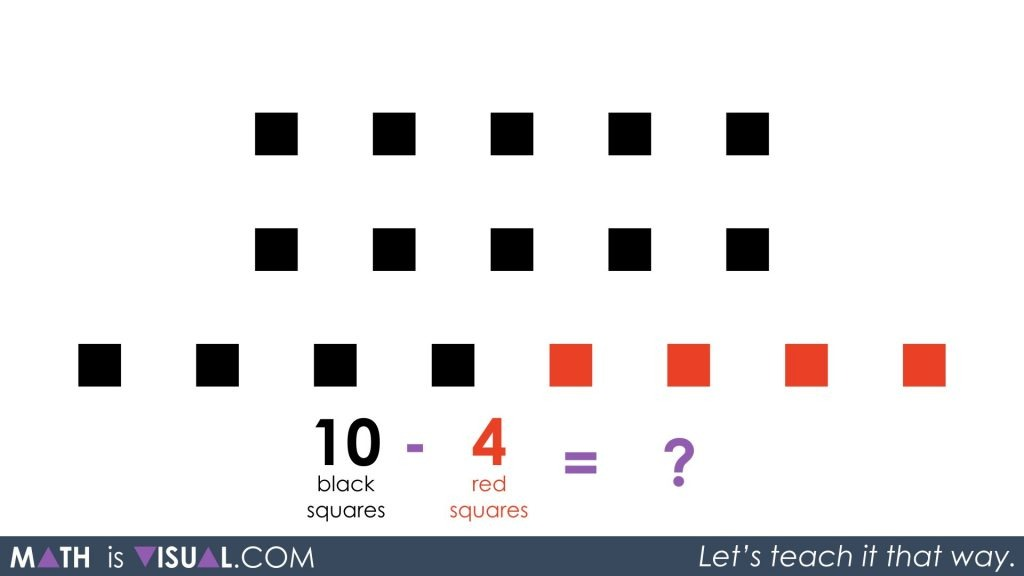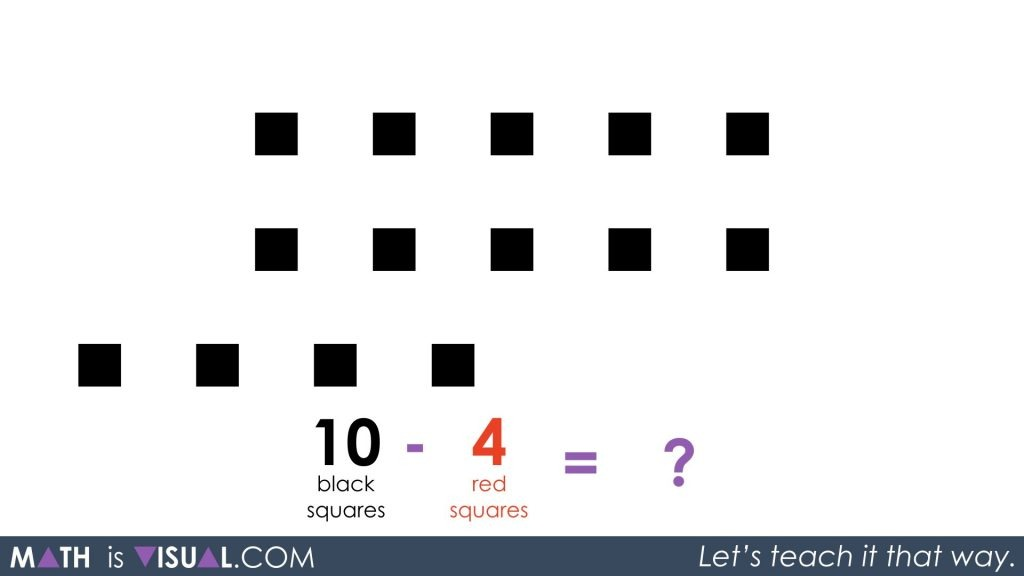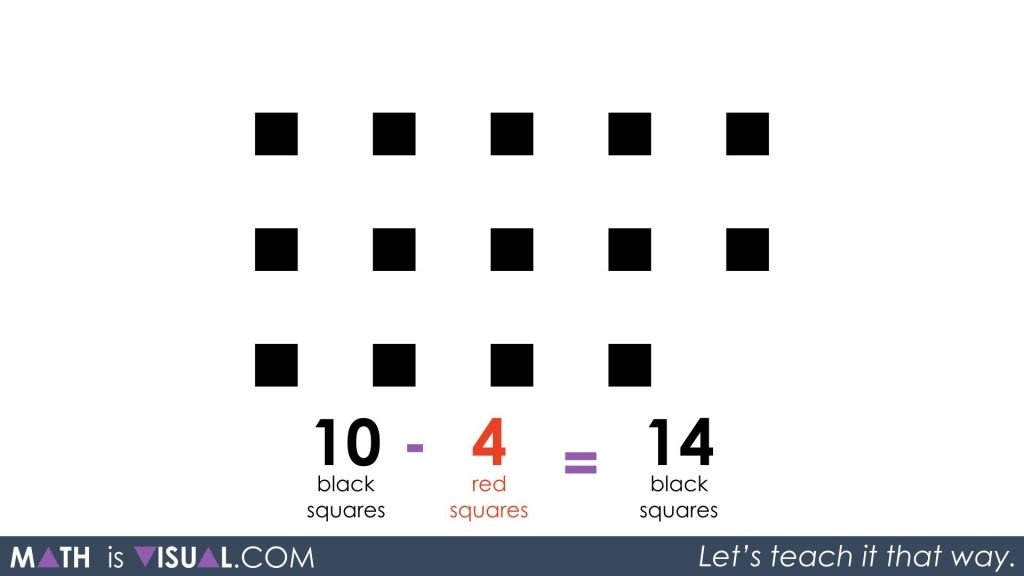In this post, we continue our work around integers from a visual perspective. You should definitely use the other integer posts first prior to attempting this task as students intuitively build an understanding progressively through each activity.

Here, we continue working with subtracting negative integers.

Math is visual. Let’s teach it that way!

## Math Is Visual Prompts

Are you finding the visual prompts useful? Leave a comment to let me know and I’ll keep them coming!

Here are a few you might consider using for your classroom.

In the Math Is Visual video, students are challenged to determine the result of:

10 black squares – 4 red squaresUsing their understanding of the zero principle as demonstrated in previous integer videos here and here, the hope is that students are developing an intuitive understanding and will add 4 red squares and 4 black squares in order to have enough red to subtract as the problem asks.After removing the 4 red squares, students should be able to see that the result is actually 10 black squares plus an additional 4 black squares, which might help point them in the direction of building a conjecture.After rearranging the concrete squares on their desks, students might have something look more like a 10 frame and 4 additional black squares.If you’re wondering whether it is worth creating some more situations on the fly like this to help your students out, the answer is: “YES!”

Give your students tons of experience with concrete and visual representations before rushing to the symbolic!

Remember: Math is visual. Let’s teach it that way!